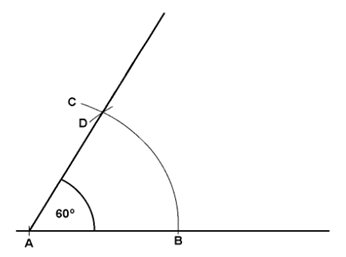# Geometry

Geometry is a branch of mathematics related to shape, size, position and properties of space. It is helpful to discover patterns, find areas, volumes, angles and length to understand the world around us. It is difficult to draw accurate geometric shapes without using geometric instruments such as ruler, compass, and set squares and so on.

As far as aviation industry is concerned, basic knowledge and understanding on this subject is sufficient. Follow the steps to construct basic geometric shapes.

### Construct two parallel lines

1. Draw a straight line.
2. Place two marks on the line.
3. Set the compass radius (r) to required distance for the parallel line.
4. Construct two arcs (a and b) at the longest reasonable distance apart on line 1.
5. Draw line 2 (parallel line) just touching the top of the arcs.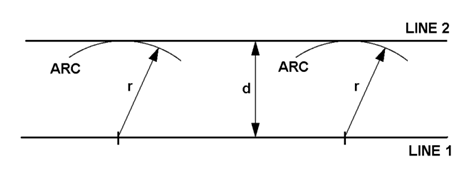### Bisect a line

1. Place the compass at one end of line segment ensuring the radius (r) is set greater than half the line length.
2. Draw arcs above and below the line, leaving the radius unaltered.
3. Draw arcs from other line end with the same compass width.
4. Draw a perpendicular line (bisecting line) through arc intersections.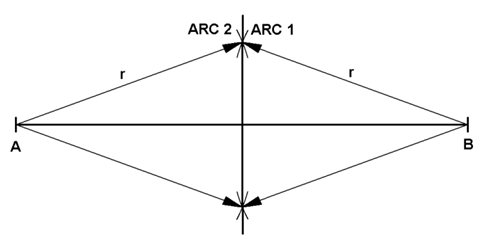### Construct a perpendicular at a point on a line

1. Set the compass on A (the place where perpendicular line is to be created) with a medium width.
2. Draw an arc on each side of A, creating two points.
3. Form the centres of two further arcs 2 and 3 with the increased compass radius.
4. Draw a line from A to the intersecting point on the top, which will be a perpendicular line.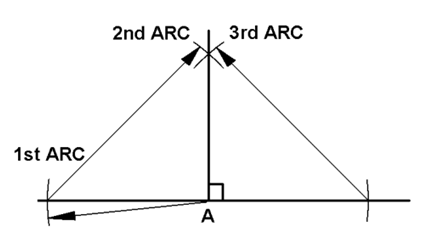### Construct a perpendicular from a point to a line

1. Set the compass point on A.
2. Set the radius greater than the distance to the line, in order to cut the line at C and D.
3. Reduce the compass radius and draw two arcs 3 and 4 from the compass points C and D.
4. Draw a line from A through the intersecting point E, which will be a perpendicular line.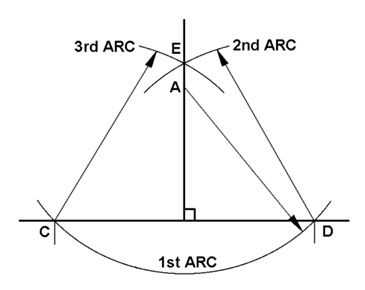### Divide a line into equal parts

1. Draw a line with A and B points.
2. Draw a second construction ray (AD) from A at an angle.
3. Place the compass on A and adjust the width depending upon the number of parts required.
4. Without changing the radius draw the arcs on the ray line. And name the final point as D.
5. Join the point D to the end of the original line B.
6. Draw parallel lines to this line from each of the stepped-off points, thus dividing the line into required number of equal parts.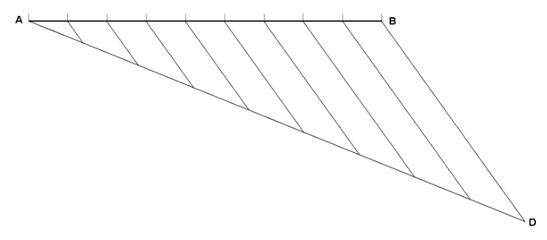### Bisect an angle CAB

1. Place the compass point on the angle’s vertex.
2. Draw an arc across each leg by setting the radius to any width.
3. Make two arcs 2 and 3 in the angle’s interior with reduced radius.
4. Draw a line from A to where the arcs cross, which bisects CAB.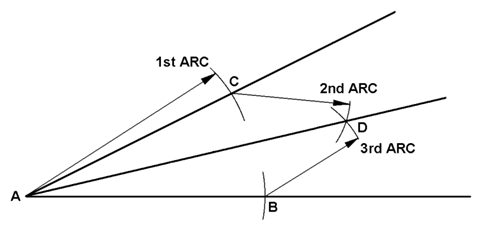### Construct a 60o angle

1. Draw a line with A and B points.
2. Set the compass width to half the length of AB and place it on the point A.
3. Draw a big arc above crossing AB line.
4. Place the compass on the crossing point and draw another arc D across the first.
5. Draw a line from A through the arc intersection point, which will form a 60o angle with AB.